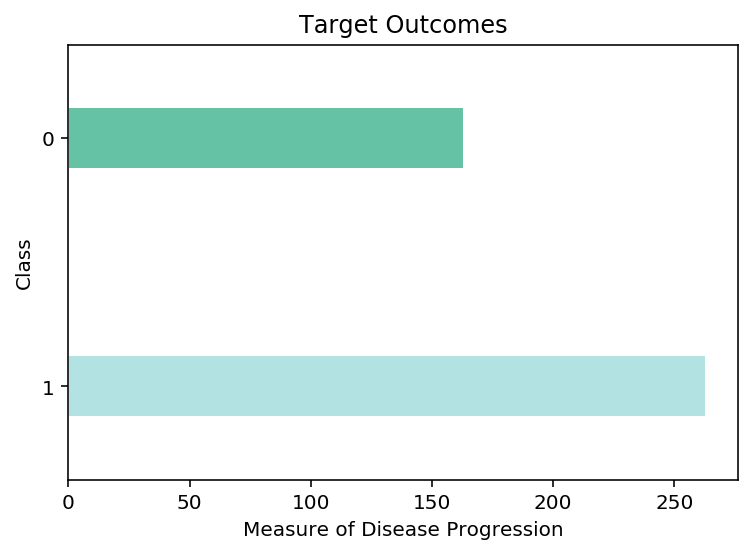22 Sep

## XG Boost Classifier

XG Boost is a pretty famous machine learning algorithm introduced in 2012. XG Boost is a gradient boosting decision tree algorithm. Instead of training a large ensemble of trees at once (like in the Random Forest Algorithm), XG Boost will train the group of trees one by one, calculating the error after building each tree, then building the next tree to reduce the previous mistake. The final predictor will be an average of all the trees.

In this notebook, I will be looking at the famous breastcancer dataset. This dataset is a multi-class classification problem, where I need to predict the correct target for each observation from a range of possible classes. We will attempt to predict the proper target class using this model, given the feature of each type of class, I often reuse this dataset between my tree-based notebooks. Using the same dataset makes it very easy to compare and contrast the performance of different tree-based models, and keep the trees a reasonable size.

Dataset

Breast Cancer Dataset: https://www.kaggle.com/hdza1991/breast-cancer-wisconsin-data-set

### Import Preliminaries¶

In :
%matplotlib inline
%config InlineBackend.figure_format='retina'

# Import modules
import matplotlib.pyplot as plt
import matplotlib as mpl
import numpy as np
import pandas as pd
import seaborn
import warnings

from sklearn.model_selection import train_test_split, cross_val_score, GridSearchCV
from xgboost import XGBClassifier

# Set pandas options
pd.set_option('max_columns',1000)
pd.set_option('max_rows',30)
pd.set_option('display.float_format', lambda x: '%.3f' % x)

# Set plotting options
mpl.rcParams['figure.figsize'] = (9.0, 3.0)

# Set warning options
warnings.filterwarnings('ignore');


### Import Data¶

In :
# Import Breast Cancer data
X, y = breast_cancer.data, breast_cancer.target

# Conduct a train-test split on the data
train_x, test_x, train_y, test_y = train_test_split(X,y)

# View the training dataframe

Out:
mean radius mean texture mean perimeter mean area mean smoothness mean compactness mean concavity mean concave points mean symmetry mean fractal dimension radius error texture error perimeter error area error smoothness error compactness error concavity error concave points error symmetry error fractal dimension error worst radius worst texture worst perimeter worst area worst smoothness worst compactness worst concavity worst concave points worst symmetry worst fractal dimension
0 13.010 22.220 82.010 526.400 0.063 0.019 0.002 0.002 0.140 0.052 0.173 1.142 1.101 14.340 0.003 0.002 0.002 0.002 0.016 0.001 14.000 29.020 88.180 608.800 0.081 0.034 0.008 0.009 0.230 0.058
1 16.780 18.800 109.300 886.300 0.089 0.092 0.084 0.066 0.189 0.055 0.599 1.391 4.129 67.340 0.006 0.025 0.026 0.016 0.021 0.003 20.050 26.300 130.700 1260.000 0.117 0.212 0.232 0.147 0.281 0.072
2 12.460 24.040 83.970 475.900 0.119 0.240 0.227 0.085 0.203 0.082 0.298 1.599 2.039 23.940 0.007 0.072 0.077 0.014 0.018 0.010 15.090 40.680 97.650 711.400 0.185 1.058 1.105 0.221 0.437 0.207
3 12.830 22.330 85.260 503.200 0.109 0.180 0.170 0.069 0.212 0.073 0.306 1.069 2.257 25.130 0.007 0.039 0.047 0.015 0.017 0.006 15.200 30.150 105.300 706.000 0.178 0.534 0.628 0.198 0.341 0.124
4 10.600 18.950 69.280 346.400 0.097 0.115 0.064 0.026 0.192 0.065 0.451 1.197 3.430 27.100 0.007 0.036 0.034 0.014 0.035 0.003 11.880 22.940 78.280 424.800 0.121 0.252 0.192 0.079 0.294 0.076

### Data Overview¶

In :
# Plot a barplot of the target clasees
pd.Series(train_y).value_counts().plot.barh(grid=False,
color=['#B2E2E2','#66C2A4'],
width=0.25,edgecolor='w')
plt.title('Target Outcomes')
plt.ylabel('Class')
plt.xlabel('Measure of Disease Progression');### Fit the Model¶

In [ ]:
# Fit the intial model
xgb_model = XGBClassifier(n_estimators=100, n_jobs=-1)
xgb_model.fit(train_x, train_y);


### Model Evaluation¶

##### Cross Validation Score¶
In [ ]:
# View the cross validation score of the intial model
scores = cross_val_score(xgb_model, train_x, train_y, cv=10,
scoring='accuracy')
print(f'Cross Validation Score: {scores.mean():.5f}')

##### Confustion Matrix¶
In [ ]:
# Training Confusion Matrix
from sklearn.metrics import confusion_matrix
cmatrix = pd.DataFrame(confusion_matrix(train_y, xgb_model.predict(train_x)))
cmatrix.index.name = 'class'

cmatrix['result'] = 'actual'
cmatrix.set_index('result', append=True, inplace=True)
cmatrix = cmatrix.reorder_levels(['result', 'class'])
cmatrix = cmatrix.stack()
cmatrix = pd.DataFrame(cmatrix)
cmatrix.columns = ['prediction']
cmatrix.unstack()

##### Feature Importance¶
In [ ]:
# Plot Tree's Feature Importance
plt.figure(figsize=(10,5))
n_features = breast_cancer.data.shape
plt.barh(range(n_features), xgb_model.feature_importances_, align='center',
color='#4D977E')
plt.yticks(np.arange(n_features), breast_cancer.feature_names)
plt.title('XGBoost Feature Importance')
plt.xlabel("Feature importance")
plt.ylabel("Features")
plt.ylim(-1, n_features);
plt.xlim(0,0.25)

##### Parameter Tuning¶
In [ ]:
# Define paraameter range and score lists
n_estimators_range = np.arange(0,300, 25)[1:]
train_score = []
test_score = []

# Train a knn_model for every neighbour value in our list
for i in n_estimators_range:
xgb_model=XGBClassifier(n_estimators = i, n_jobs=-1).fit(train_x,train_y)
train_score.append(cross_val_score(xgb_model, train_x,
train_y, cv=10,
scoring='accuracy').mean())
test_score.append(cross_val_score(xgb_model, test_x,
test_y, cv=10,
scoring='accuracy').mean())
# Plot our results
mpl.rcParams['figure.figsize'] = (9.0, 6.0)
plt.plot(n_estimators_range,train_score,label="Train",linewidth=2, color='#66C2A4')
plt.plot(n_estimators_range,test_score,label="Test", linewidth=2,linestyle='--', color='#B2E2E2')
plt.legend()
plt.title('Random Forest Model')
plt.xlabel('Number of Estimators')
plt.ylabel('Accuracy');

##### Grid Searching Turnning¶
In [ ]:
# Set up parameter grid
grid = {'n_estimators':np.arange(0,100, 25)[1:],
'learning_rate': [0.01, 0.025, 0.05,0.005],
'max_depth':list(range(2,30,4)),
'booster': ['gbtree','gblinear','dart'],
'reg_alpha':[0,1]}

# Conduct gird search
grid_search = GridSearchCV(estimator=xgb_model, param_grid=grid,
scoring='accuracy', n_jobs=-1, refit=True, cv=10,
return_train_score=True)
# Fit model
grid_search.fit(train_x,train_y);

# Print out the parameter for the best score
print('Accuracy of best parameters: %.5f'%grid_search.best_score_)
print('Best parameters: %s' %grid_search.best_params_)


### Final Model¶

In [ ]:
# Fit the final model
xgb_model = XGBClassifier(booster='gbtree', learning_rate=0.05,
max_depth=6, n_estimators = 75)
xgb_model.fit(train_x, train_y)

# View the cross validation score of the intial model
scores = cross_val_score(xgb_model, train_x, train_y, cv=10,
scoring='accuracy')
print(f'Cross Validation Score: {scores.mean():.5f}')

##### Confustion Matrix¶
In [ ]:
# Training confusion matrix
from sklearn.metrics import confusion_matrix
cmatrix = pd.DataFrame(confusion_matrix(train_y, xgb_model.predict(train_x)))
cmatrix.index.name = 'class'

cmatrix['result'] = 'actual'
cmatrix.set_index('result', append=True, inplace=True)
cmatrix = cmatrix.reorder_levels(['result', 'class'])
cmatrix = cmatrix.stack()
cmatrix = pd.DataFrame(cmatrix)
cmatrix.columns = ['prediction']
cmatrix.unstack()

##### Feature Importance¶
In [ ]:
# Plot ensembles's feature importance
plt.figure(figsize=(10,5))
n_features = breast_cancer.data.shape
plt.barh(range(n_features), xgb_model.feature_importances_, align='center',
color='#4D977E')
plt.yticks(np.arange(n_features), breast_cancer.feature_names)
plt.title('Random Forest Feature Importance')
plt.xlabel("Feature importance")
plt.ylabel("Features")
plt.ylim(-1, n_features)
plt.xlim(0,0.25);


### Predict Results¶

In [ ]:
# Predict the results from our test data